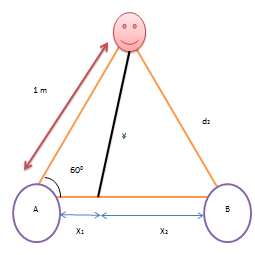# The drawing shows a loudspeaker A and point C, where a listener is positioned. A second...

## Question:

The loudspeaker {eq}A {/eq} and point {eq}C {/eq}, where a listener is positioned(1 m) at 60°. A second loudspeaker {eq}B {/eq} is located somewhere to the right of loudspeaker {eq}A {/eq}. Both speakers vibrate in phase and are playing a {eq}64.6-Hz {/eq} tone. The speed of sound is {eq}343 \frac{m}{s} {/eq}. What is the closest to speaker {eq}A {/eq} that speaker {eq}B {/eq} can be located, so that the listener hears no sound?

## Destructive Interference

The sound waves cancel out when the path difference between them is integral odd multiples of wavelength and waves amplify when the difference is even multiples.

## Answer and Explanation:

The schematic diagram is shown below (in accordance with the problem),Interference at point C must be destructive in order to hear no sound.

Mathematically,

{eq}d_2 -d_1 = \dfrac{n \lambda}{2}, \;\; n = 1, 3, 5... {/eq}

We know that {eq}v_{(wave)} = \lambda f\\ where,\\ v_{(wave)} = velocity\\ \lambda = wavelength\\ f = frequency {/eq}

Plugin the given values we get,

{eq}v_{(wave)} = \lambda f\\ \lambda = \dfrac{343}{64.6}\\ \lambda = 9.91\;m {/eq}

For minimum distance, n = 1 so,

{eq}d_2 = \dfrac{n \lambda}{2}+d_1\\ d_2 = \dfrac{9.91}{2}+1 = 5.95\;m {/eq}

Therefore,

{eq}x_1 = (1) \cos60° = 0.500\; m\\ y = (1) \sin60° = 0.866\;m {/eq}

Thus,

{eq}x^2_2 +y^2 = d^2_2\\ d_2 = \sqrt{(5.95)^2+(0.866)^2}\\ d_2 = \sqrt{35.40 - 0.749}\\ d_2 = 5.88\;m {/eq}

So the minimum distance will be,

{eq}x_1 +x_2 = 0.500+5.88 = 6.38\;m {/eq}